# Ellipsoidal coordinates

spatial elliptic coordinates

The numbers,andconnected with Cartesian rectangular coordinates,andby the formulas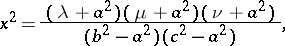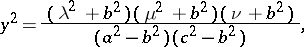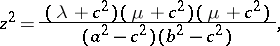where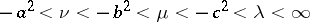. The coordinate surfaces are (see Fig.): ellipses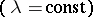, one-sheet hyperbolas (), and two-sheet hyperbolas (), with centres at the coordinate origin.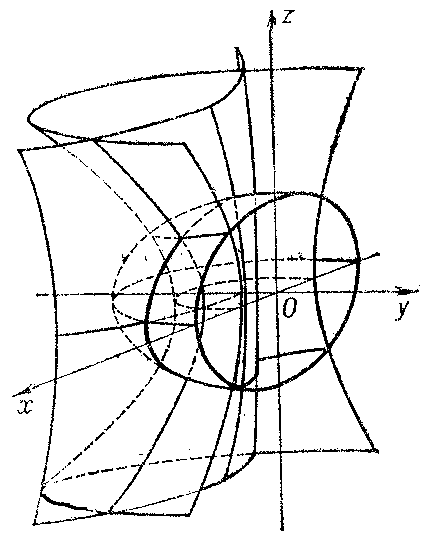Figure: e035420a

The system of ellipsoidal coordinates is orthogonal. To every triple of numbers,andcorrespond 8 points (one in each octant), which are symmetric to each other relative to the coordinate planes of the system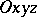.

The Lamé coefficients are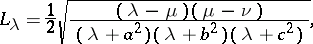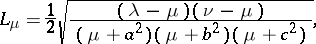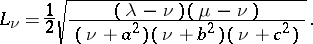If one of the conditions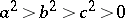in the definition of ellipsoidal coordinates is replaced by an equality, then degenerate ellipsoidal coordinate systems are obtained.